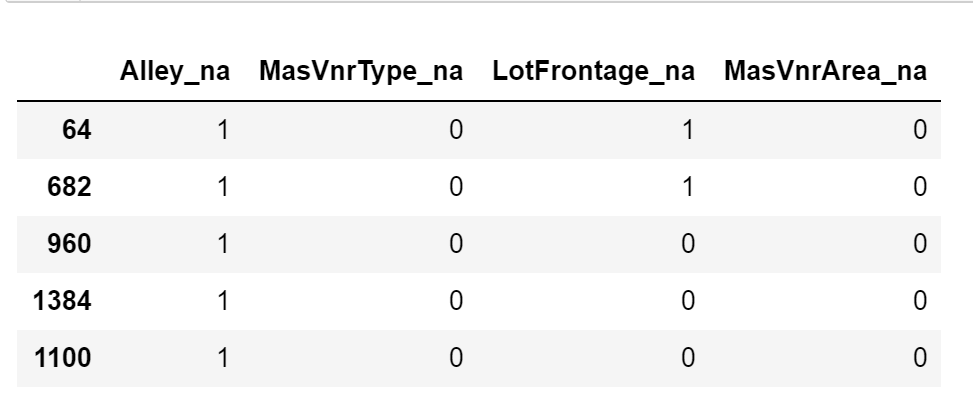The `AddMissingIndicator()` adds a binary variable indicating if observations are missing (missing indicator). It adds missing indicators to both categorical and numerical variables.

You can select the variables for which the missing indicators should be created passing a variable list to the `variables` parameter. Alternatively, the imputer will automatically select all variables.

The imputer has the option to add missing indicators to all variables or only to those that have missing data in the train set. You can change the behaviour using the parameter `missing_only`.

If `missing_only=True`, missing indicators will be added only to those variables with missing data in the train set. This means that if you passed a variable list to `variables` and some of those variables did not have missing data, no missing indicators will be added to them. If it is paramount that all variables in your list get their missing indicators, make sure to set `missing_only=False`.

It is recommended to use `missing_only=True` when not passing a list of variables to impute.

Below a code example using the House Prices Dataset (more details about the dataset here).

First, let’s load the data and separate it into train and test:

```import numpy as np
import pandas as pd
import matplotlib.pyplot as plt
from sklearn.model_selection import train_test_split

# Separate into train and test sets
X_train, X_test, y_train, y_test = train_test_split(
data.drop(['Id', 'SalePrice'], axis=1), data['SalePrice'], test_size=0.3, random_state=0)
```

Now we set up the imputer to add missing indicators to the 4 indicated variables:

```# set up the imputer
variables=['Alley', 'MasVnrType', 'LotFrontage', 'MasVnrArea'],
)

# fit the imputer
```

Because we left the default value for `missing_only`, the `AddMissingIndicator()` will check if the variables indicated above have missing data in X_train. If they do, missing indicators will be added for all 4 variables looking forward. If one of them had not had missing data in X_train, missing indicators would have been added to the remaining 3 variables only.

We can know which variables will have missing indicators by looking at the variable list in the `AddMissingIndicator()`’s attribute `variables_`.

```# transform the data

```Note that after adding missing indicators, we still need to replace NA in the original variables if we plan to use them to train machine learning models.

## Tip#

Missing indicators are commonly used together with random sampling, mean or median imputation, or frequent category imputation.

## More details#

In the following Jupyter notebook you will find more details on the functionality of the `AddMissingIndicator()`, including how to use the parameter `missing_indicator` and how to select the variables automatically.

All notebooks can be found in a dedicated repository.